# Playing Hide and Seek

## OBJECTIVE: To learn how to multiply decimals

In a previous lesson, you learned how to ADD and SUBTRACT decimals. Lining 'Em Up: https://www.geogebra.org/m/xe7bhcvz In this lesson, you're going to learn how to MULTIPLY decimals. Follow this simple procedure: First, ignore the decimal points, then proceed with multiplication, and finally put back the decimal point so that the number of decimal places in the product will equal the number of decimal places in the multiplicand and the multiplier put together. Examples: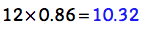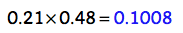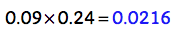The applet below provides practice for multiplying decimals. You may change the maximum value by typing a new maximum value into the entry box. Solve the problem first on a separate sheet of paper, then type in your answer. The applet will show the correct answer up to two decimal places. Repeat as many times as needed to master the concept.
Sometimes, you'll be asked to multiply a decimal by powers of 10 (e.g., 10, 100, 1000, etc.). To perform this multiplication, simply move the decimal point to the right the same number of places as there are zeros in the multiplier. Fill in any empty spaces that will appear with zeros. Examples: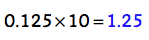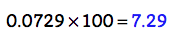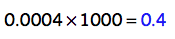Below is a set of problems that require you to multiply decimals by powers of 10.

## In this lesson, you learned how to multiply decimals.

In a future lesson, you'll learn how to divide decimals. Hope you ENJOYED today's lesson!